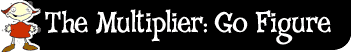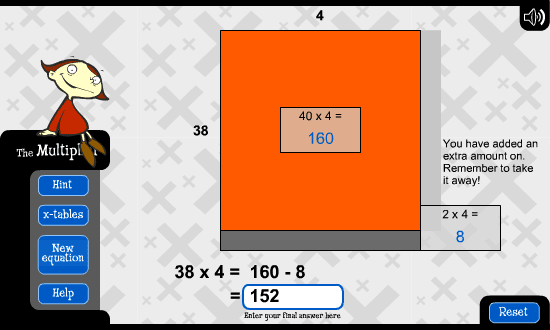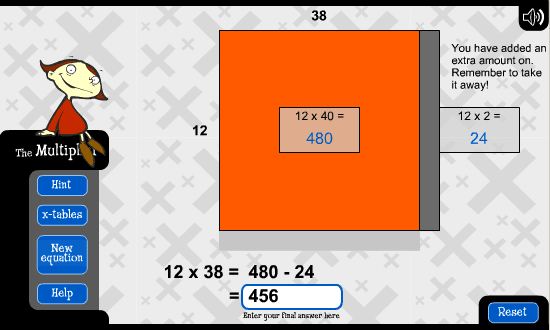﻿ The multiplier: go figure: making tens# Making tens

The idea behind this strategy is that you round up one of the numbers to the nearest ten. Then you can use your 10 times table to help work out the answer. Don't forget to take away the extra numbers that were added when you rounded up.

Let's look at 38 times 4. I round 38 to 40. 40 times 4 is 160. I added 2 before so now I take away 2 times 4, which is 8, to give me an answer of 152.Let's use this strategy to work out 9 times 17.

10 times 17 is 170. Take away 1 times 17. My answer is 153.

I could use the same strategy for a harder multiplication like 12 times 38.I could round 38 up to 40, because I know that 12 times 40 is 480. I then take away 2 times 12, which is 24. 480 take away 24 equals 456.

Let's use this strategy again to work out 28 times 17.